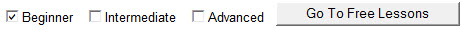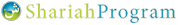Proof By Induction

# Induction & Deduction

There are two major types of air-tight proof, generally speaking: induction and deduction. Induction is the process of exhaustively surveying the entire class about which we are making a claim in order to show that the claim applies to all members. For example, if we wanted to inductively show that all mules are sterile, we’d have to survey all mules and show that each one individually is sterile. This type of proof is obviously only feasible in cases where the class is relatively small. We would never be able to prove anything about classes of infinite size. Moreover, we would never be able to prove anything about classes some of whose members no longer exist, as how many mules lived once but live no longer. Furthermore, we would have to keep the process of proof perpetual for classes where new members are continuously introduced, as how new mules are born every day. Clearly, induction is employed in very particular cases.

The other major type of proof is deduction. This is actually an entire family of proofs; any air-tight method of proof that is not induction is called deduction. More specifically, deductive proofs are those in which we use logical reasoning to establish a claim. We use axioms, other proofs, and logical mechanisms to establish the claim.

We’ve already seen many types of deductive proof. One is the Syllogism, where we use the relationship between three classes to extend a ruling from one to another. Such proofs can be reviewed at the Proofs tutorial. Yet another type of proof is called Proof by Contradiction, where we prove a statement by assuming its opposite to be true and looking for contradictions caused by that assumption.

# Mathematical Induction

Here we introduce yet another method of deductive proof known as Mathematical Induction. This is often abbreviated to just Induction and should not be confused with the type of induction mentioned hereinabove – when we use the term Induction, we are referring to Mathematical Induction for the duration of this article.

All of the proofs we have studied thus far – such as proving conjunctive statements, proving disjunctive statements, proving conditional statements, syllogisms, proof by contradiction – and some we have not studied, are all pretty basic types of proof. Induction, on the other hand, is considered quite advanced and is very, very powerful. Although used primarily in math and science, it does have other applications.

Proving a claim by induction involves three steps:

1.       show that your claim is true for the very first member of the class

2.       assume it is true for some random member K in the class

3.       using that assumption, show that it is true for the next member, K + 1

# Example

Given a bunch of squares of any size, is it always possible to dissect the squares somehow and combine them to form one large square? Let’s say we have 15 squares where some have length 2cm, others have length 32cm, and so forth. Can we combine these squares to form one big square just by dissection? Note: dissecting a square means to pick two points on its perimeter and draw a line between them in order to split them into two (possibly unequal) shapes. The answer is Yes. But it is not at all obvious, so let’s prove this by induction.

STEP 1: The first step in the inductive process is to show that the claim holds true for the first member. In our case, this means showing that two squares can be dissected and combined to form one larger square. (Notice that the first step was not to demonstrate the claim for one square because this claim is vacuously true for only one square.) The solution to this can be seen here.

STEP 2: The next step in the inductive process is to assume the claim holds for the K-th member. In our case, this means that we assume that we can take K squares of any size and dissect and combine them to form one large square. So let’s just assume that.

STEP 3: The final leg of the proof involves using the assumption from STEP 2 and proving our claim for the K + 1 member. For this example, that means we need to show that we can take K + 1 squares of any size and dissect and combine them to form one large square. Well, recall from STEP 1 that we can take any two squares and dissect and combine them to form one square. So let’s do this with any two of our K + 1 squares. Now we are left with only K squares because we combined two of them. But recall from STEP 2 that any K squares can be dissected and combined to form one large square. And there we have it.

# Why It Works

There is no proof of veracity for the Principle of Mathematical Induction; it is axiomatic. However we can give an intuitive understanding of why it might be valid. What we are doing in induction is assuming that our claim is true for the K-th member. We then show that by moving on to the next member, K + 1, nothing really changes; our claim is still true and adding the next member does not change that. Consider the example we worked on above. We assumed that K squares can be combined to form one square. We then showed that adding another square into the mix does nothing to change that fact; it is still true despite that new square. And in step 1 we showed that our claim is true for two squares. Since our claim is true for two squares and adding a square does nothing to change that, it’s true for three squares. Since adding a square does nothing to change that, it’s true for four squares, and so on and so on ad infinitum.

# Condition for Using Mathematical Induction

Induction is a very powerful tool, especially when dealing with classes of infinite size. There is a condition to using this, however. In order to be able to use induction to prove a claim on a class, the class needs to be Well-Ordered. This means two things:

1.       It must be possible to order the members of the class somehow. In our example above, the order happened to be based on the number of squares we were dealing with.

2.       Once ordered somehow, the class must have a smallest element. In our example, the smallest element was two squares. Notice, however, that the class does NOT need to have a largest element.